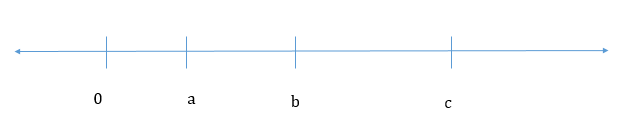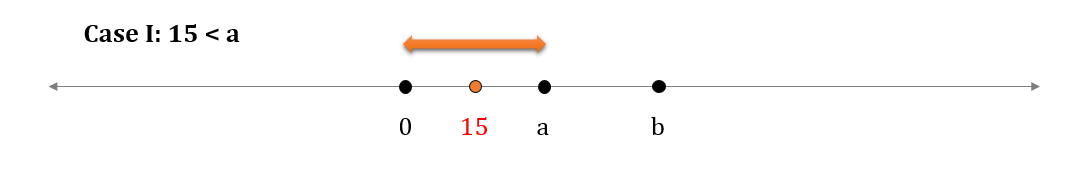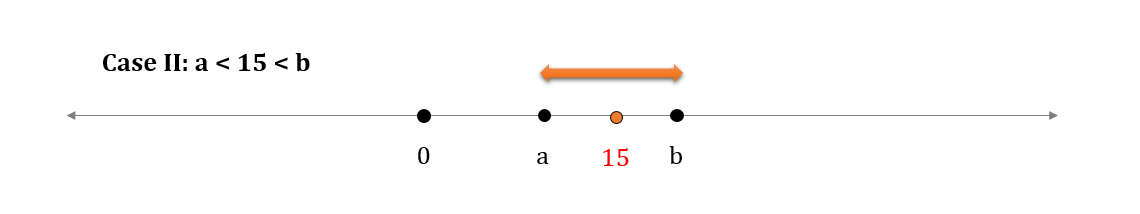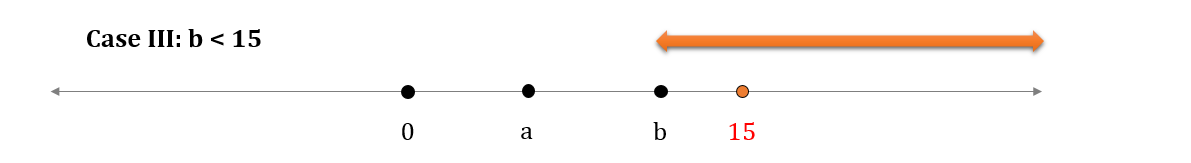GMAT Question of the Day: Daily via email | Daily via Instagram New to GMAT Club? Watch this Video

 It is currently 20 Jan 2020, 13:49### GMAT Club Daily Prep

#### Thank you for using the timer - this advanced tool can estimate your performance and suggest more practice questions. We have subscribed you to Daily Prep Questions via email.

Customized
for You

we will pick new questions that match your level based on your Timer History

Track

every week, we’ll send you an estimated GMAT score based on your performance

Practice
Pays

we will pick new questions that match your level based on your Timer History

#### Not interested in getting valuable practice questions and articles delivered to your email? No problem, unsubscribe here.# On the number line shown, the distance between 0 and a . . .

Author Message
TAGS:

### Hide Tags

e-GMAT RepresentativeV
Joined: 04 Jan 2015
Posts: 3209
On the number line shown, the distance between 0 and a . . .  [#permalink]

### Show Tags

4300:00

Difficulty:95% (hard)

Question Stats:35% (03:17) correct65% (03:17) wrongbased on 341 sessions

### HideShow timer StatisticsOn the number line shown, the distance between 0 and a, a and b, and a and c is in the ratio of 1:2:3. If the distance of point b from 15 is twice the distance of point a from 15, what is the value of |c|?

A. 9
B. 27
C. 36
D. 45
E. 54

Take a stab at this fresh question from e-GMAT. Post your analysis below.

To solve question of the week:Question of the Week

Official Solution to be provided after receiving some good analyses._________________

Originally posted by EgmatQuantExpert on 08 Nov 2016, 05:04.
Last edited by EgmatQuantExpert on 07 Aug 2018, 04:27, edited 7 times in total.
e-GMAT RepresentativeV
Joined: 04 Jan 2015
Posts: 3209
Re: On the number line shown, the distance between 0 and a . . .  [#permalink]

### Show Tags

5
2
Alright, let's look at the detailed solution of the above question. Let's use the concept of absolute value $$|p-q|$$ as the distance between the point p and q on the number line to solve this question.

Given
The following information is given in the question.
• Points a, b and c such that $$c > b > a$$
• $$a: (b – a) : (c – a) = 1: 2: 3$$
• $$|b – 15| = 2 * |a-15|$$ (We are taking the absolute values here, as we do not know the position of 15 with respect to a and b. For example, if $$b > 15$$, then $$|b – 15| = b – 15$$ but if b < 15, then$$|b – 15| = (15 - b)$$

Let’s assume the value of a to be x.
Hence, $$b-a = 2x$$ and substituting the value of a, we get the value of $$b = 3x$$
Similarly, the value of $$c = 4x$$

To find
As, we need to find |c|, which is the distance of c from the origin, we can say that $$|c| = 4x$$

Approach and Working Out
Now, we are given the relationship between the distance of points a and b with respect to 15. However, we do not know the position of 15 with respect to a and b on the number line. So, the following cases can occur depending upon the position of 15 on the number line:o Distance between 15 and $$a = a – 15 = x – 15$$ (as a is greater than 15)
o Distance between 15 and b = $$b – 15 = 3x – 15$$ (as b is greater than 15)
o $$3x – 15 = 2*(x – 15)$$ {as $$|b – 15| = 2 * |a-15|$$}
o $$3x – 15 = 2x – 30$$
o $$x = -15$$, which is not possible as x is the coordinate of a point that lies on the right hand side of 0, i.e. it is positive. So, $$x > 0$$.
o Thus, we can reject this case.o Distance between 15 and a = $$15 – a = 15 – x$$ (as 15 is greater than a)
o Distance between 15 and b = $$b – 15 = 3x – 15$$ (as b is greater than 15)
o $$3x – 15 = 2*(15 – x)$${as $$|b – 15| = 2 * |a-15|$$}
o $$3x – 15 = 30 – 2x$$
o $$5x = 45$$, i.e. $$x = 9$$.
o In this case we have assumed that $$a < 15$$. So, $$x < 15$$ (As the coordinate of point a is x). Also $$x > 0$$, since it is the coordinate of a point that lies on the right hand side of 0.
o So, $$0 < x< 15$$. As we have calculated $$x = 9$$, this is a possible case
o We have calculated above, the value of $$c = 4x$$. So, we have $$4x = 36$$o Distance between 15 and a = $$15 – a = 15 – x$$ (as 15 is greater than a)
o Distance between 15 and b = $$15 – b = 15 – 3x$$ (as 15 is greater than b)
o $$15 – 3x = 2*( 15 – x)$$ {as $$|b – 15| = 2 * |a-15|$$ }
o $$15 – 3x = 30 – 2x$$, i.e. $$x = -15$$,
o We know that $$x > 0$$. So, $$x = -15$$ is not a valid value.
o Thus, we can reject this case.

Therefore, the value of |c|is 36_________________

Originally posted by EgmatQuantExpert on 28 Nov 2016, 06:25.
Last edited by EgmatQuantExpert on 04 Dec 2016, 23:50, edited 4 times in total.
InternS
Status: I am not giving up. Not yet.
Joined: 23 Jul 2015
Posts: 40
Re: On the number line shown, the distance between 0 and a . . .  [#permalink]

### Show Tags

9
1
3
Let Point a-'x" units away from 0
Point b- 3x units away from 0
Point C- 4x units away from 0.

15 has to be between points a and b such that
3x-15=2(15-x)
=> x=9
Point c=4x=4*9=36.

Hence C

+1 Kudos to show your appreciation.
_________________
Cheers
PeeKay
##### General Discussion
ManagerS
Joined: 05 Apr 2014
Posts: 136
Location: India
Schools: ISB '19, Fox"19
GMAT 1: 660 Q48 V33
GPA: 3
Re: On the number line shown, the distance between 0 and a . . .  [#permalink]

### Show Tags

Let dist between a and 0 be x,then that between a and b would be 2x,and between b and C would be x.
Mod b-15 = 2* Mod 15-a
So, x= 9
Therefore mod c = 27

Sent from my HM 1SW using GMAT Club Forum mobile app
InternB
Joined: 19 Nov 2016
Posts: 9
Re: On the number line shown, the distance between 0 and a . . .  [#permalink]

### Show Tags

5
1
From: "The distance between 0 and a, a and b, and a and c is in the ratio of 1:2:3"
Set a= 1, we have b = 3 and c =4
=> a : b : c is in the ratio of 1 : 3 : 4
=> c is divisible by 4
=> c is 36
C
e-GMAT RepresentativeV
Joined: 04 Jan 2015
Posts: 3209
Re: On the number line shown, the distance between 0 and a . . .  [#permalink]

### Show Tags

1
nailin16 wrote:
Let dist between a and 0 be x,then that between a and b would be 2x,and between b and C would be x.
Mod b-15 = 2* Mod 15-a
So, x= 9
Therefore mod c = 27

Sent from my HM 1SW using GMAT Club Forum mobile app

You have correctly calculated the value of x i.e. the distance between point a and 0 on the number line.
However, the value of |c| is 4x, and hence the answer would be 36.

Kindly review our solution above to understand where you went wrong.
_________________
e-GMAT RepresentativeV
Joined: 04 Jan 2015
Posts: 3209
Re: On the number line shown, the distance between 0 and a . . .  [#permalink]

### Show Tags

3
khiemchii wrote:
From: "The distance between 0 and a, a and b, and a and c is in the ratio of 1:2:3"
Set a= 1, we have b = 3 and c =4
=> a : b : c is in the ratio of 1 : 3 : 4
=> c is divisible by 4
=> c is 36
C

There is a small flaw in your approach. You seem to have assumed c as a multiple of 4, which may not be the case always. For instance, let's look at a slightly different version of the same question.

On the number line shown, the distance between 0 and a, a and b, and a and c is in the ratio of 1:2:3. If the distance of point b from 11.25 is twice the distance of point a from 15, what is the value of |c|?

A. 9
B. 27
C. 36
D. 45
E. 54

Solve this question using the approach mentioned by you and also using the solution provided by us. If you use the approach mentioned by you in your response, you will still the answer as 36 (Choice C). However, the answer to this modified version would be 27 (choice B), which is clearly not a multiple of 4._________________
InternJoined: 15 Nov 2015
Posts: 23
Location: Netherlands
Schools: HEC Dec '17 (II)
GMAT 1: 730 Q47 V44
GPA: 3.99
Re: On the number line shown, the distance between 0 and a . . .  [#permalink]

### Show Tags

EgmatQuantExpert wrote:If the distance of point b from 15 is twice the distance of point a from 15

In your previous explanation you have also placed the point "15" to the left of A and to the right of B, but would this even be possible given the above limitation? Given the order of the letters, the only way I can see this condition being met is if 15 is 1/3 on the line between A and B.

e-GMAT RepresentativeV
Joined: 04 Jan 2015
Posts: 3209
Re: On the number line shown, the distance between 0 and a . . .  [#permalink]

### Show Tags

koenh wrote:
EgmatQuantExpert wrote:If the distance of point b from 15 is twice the distance of point a from 15

In your previous explanation you have also placed the point "15" to the left of A and to the right of B, but would this even be possible given the above limitation? Given the order of the letters, the only way I can see this condition being met is if 15 is 1/3 on the line between A and B.

Yes, you're absolutely right.We have taken these cases as Case I (15 to the left of a or a>15) and Case III (15 to the right of B, 15>b). However, if you go through our explanation in details, you will understand that we have also concluded that these cases are not possible. Hence, the only case possible is Case II, in which 15 lies between a and b. The same has been explained in details in the solution.

The reason why we have discussed all possibilities is to ensure that we critically analyze all cases before arriving at a conclusion. Doing so, is extremely important while solving GMAT questions.

Regards,
Saquib
_________________
GMATH TeacherP
Status: GMATH founder
Joined: 12 Oct 2010
Posts: 935
Re: On the number line shown, the distance between 0 and a . . .  [#permalink]

### Show Tags

EgmatQuantExpert wrote:
On the number line shown, the distance between 0 and a, a and b, and a and c is in the ratio of 1:2:3. If the distance of point b from 15 is twice the distance of point a from 15, what is the value of |c|?

A. 9
B. 27
C. 36
D. 45
E. 54

Using info given (figure included) we have DATA and FOCUS as below:

$$0 < a < b < c\,\,\left( * \right)$$

$${\text{dist}}\left( {0,a} \right) = a$$

$$\left. \begin{gathered} {\text{dist}}\left( {a,b} \right) = 2a\, \hfill \\ {\text{dist}}\left( {a,c} \right) = 3a \hfill \\ \end{gathered} \right\}\,\,\,\mathop \Rightarrow \limits^{\left( * \right)} \,\,\,{\text{dist}}\left( {b,c} \right) = 3a - 2a = a$$

$$? = c = 4a$$

From the fact that $${\text{dist}}\left( {b,15} \right) = 2 \cdot {\text{dist}}\left( {a,15} \right)$$ we have three possible scenarios:

$$b < 15:$$

$$15 - b = 2\left( {15 - a} \right)\,\,\,\, \Rightarrow \,\,\,\,15 = 2a - b = 2a - 3a = - a\,\,\,\, \Rightarrow \,\,\,a < 0\,\,\,\,{\text{impossible}}\,\,$$

$$a < 15 \leqslant b:$$

$$b - 15 = 2\left( {15 - a} \right)\,\,\,\, \Rightarrow \,\,\,\,3 \cdot 15 = 2a + b = 2a + 3a = 5a\,\,\,\, \Rightarrow \,\,? = 4a = 4 \cdot 9 = 36$$

And from the fact that we have already found (one viable) correct alternative choice, no need to evaluate the third scenario!

This solution follows the notations and rationale taught in the GMATH method.

Regards,
Fabio.
Attachments18Set18_3w.gif [ 1.92 KiB | Viewed 2584 times ]

_________________
Fabio Skilnik :: GMATH method creator (Math for the GMAT)
Our high-level "quant" preparation starts here: https://gmath.net
InternB
Joined: 27 Apr 2018
Posts: 17
Location: India
Schools: CBS '21, Marshall '21
GMAT 1: 600 Q44 V29
GPA: 3.9
Re: On the number line shown, the distance between 0 and a . . .  [#permalink]

### Show Tags

EgmatQuantExpert wrote:
koenh wrote:
EgmatQuantExpert wrote:If the distance of point b from 15 is twice the distance of point a from 15

In your previous explanation you have also placed the point "15" to the left of A and to the right of B, but would this even be possible given the above limitation? Given the order of the letters, the only way I can see this condition being met is if 15 is 1/3 on the line between A and B.

Yes, you're absolutely right.We have taken these cases as Case I (15 to the left of a or a>15) and Case III (15 to the right of B, 15>b). However, if you go through our explanation in details, you will understand that we have also concluded that these cases are not possible. Hence, the only case possible is Case II, in which 15 lies between a and b. The same has been explained in details in the solution.

The reason why we have discussed all possibilities is to ensure that we critically analyze all cases before arriving at a conclusion. Doing so, is extremely important while solving GMAT questions.

Regards,
Saquib

Hello!! With all respect, I request you to explain how you got C=4x ?? I am getting a lil bit confused here.
InternB
Joined: 12 Aug 2019
Posts: 19
Location: United States
GMAT 1: 690 Q49 V33
Re: On the number line shown, the distance between 0 and a . . .  [#permalink]

### Show Tags

EgmatQuantExpert wrote:
Alright, let's look at the detailed solution of the above question. Let's use the concept of absolute value $$|p-q|$$ as the distance between the point p and q on the number line to solve this question.

Given
The following information is given in the question.
• Points a, b and c such that $$c > b > a$$
• $$a: (b – a) : (c – a) = 1: 2: 3$$
• $$|b – 15| = 2 * |a-15|$$ (We are taking the absolute values here, as we do not know the position of 15 with respect to a and b. For example, if $$b > 15$$, then $$|b – 15| = b – 15$$ but if b < 15, then$$|b – 15| = (15 - b)$$

Let’s assume the value of a to be x.
Hence, $$b-a = 2x$$ and substituting the value of a, we get the value of $$b = 3x$$
Similarly, the value of $$c = 4x$$

To find

As, we need to find |c|, which is the distance of c from the origin, we can say that $$|c| = 4x$$

Approach and Working Out
Now, we are given the relationship between the distance of points a and b with respect to 15. However, we do not know the position of 15 with respect to a and b on the number line. So, the following cases can occur depending upon the position of 15 on the number line:o Distance between 15 and $$a = a – 15 = x – 15$$ (as a is greater than 15)
o Distance between 15 and b = $$b – 15 = 3x – 15$$ (as b is greater than 15)
o $$3x – 15 = 2*(x – 15)$$ {as $$|b – 15| = 2 * |a-15|$$}
o $$3x – 15 = 2x – 30$$
o $$x = -15$$, which is not possible as x is the coordinate of a point that lies on the right hand side of 0, i.e. it is positive. So, $$x > 0$$.
o Thus, we can reject this case.o Distance between 15 and a = $$15 – a = 15 – x$$ (as 15 is greater than a)
o Distance between 15 and b = $$b – 15 = 3x – 15$$ (as b is greater than 15)
o $$3x – 15 = 2*(15 – x)$${as $$|b – 15| = 2 * |a-15|$$}
o $$3x – 15 = 30 – 2x$$
o $$5x = 45$$, i.e. $$x = 9$$.
o In this case we have assumed that $$a < 15$$. So, $$x < 15$$ (As the coordinate of point a is x). Also $$x > 0$$, since it is the coordinate of a point that lies on the right hand side of 0.
o So, $$0 < x< 15$$. As we have calculated $$x = 9$$, this is a possible case
o We have calculated above, the value of $$c = 4x$$. So, we have $$4x = 36$$o Distance between 15 and a = $$15 – a = 15 – x$$ (as 15 is greater than a)
o Distance between 15 and b = $$15 – b = 15 – 3x$$ (as 15 is greater than b)
o $$15 – 3x = 2*( 15 – x)$$ {as $$|b – 15| = 2 * |a-15|$$ }
o $$15 – 3x = 30 – 2x$$, i.e. $$x = -15$$,
o We know that $$x > 0$$. So, $$x = -15$$ is not a valid value.
o Thus, we can reject this case.

Therefore, the value of |c|is 36after we establish c = 4x...can we just look at the answer choices for multiples of 4 and narrow down to choice CRe: On the number line shown, the distance between 0 and a . . .   [#permalink] 01 Nov 2019, 19:03
Display posts from previous: Sort by

# On the number line shown, the distance between 0 and a . . .